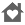MatroidEveryone
32
Matroid is the first application of matrices for Android that lets you:

» Add, subtract and multiply matrices.
» Calculate determinants, ranges and inverse matrices.
» Delete rows and columns linearly dependent of a matrix.
» Solve systems of linear equations determined and undetermined, by obtaining their solutions based on parameters if necessary.

And all of this, always working with both fractions and decimal numbers of completely arbitrary precision to obtain results as accurate as desired.
Furthermore, Matroid has no limits on the size of matrices!

This application is constantly evolving, and it is anticipated in future updates calculating characteristic polynomials and eigenvalues.

In this free version of the application, options for multiplying matrices, get the inverse and solve systems of equations are not available.
Collapse

Review Policy
4.4
32 total
5
4
3
2
1

What's New

» Add, subtract and multiply matrices.
» Calculate determinants, ranges and inverse matrices.
» Delete rows and columns linearly dependent of a matrix.
» Solve systems of linear equations determined and undetermined, by obtaining their solutions based on parameters if necessary.
Collapse

Eligible for Family LibraryEligible if bought after 7/2/2016. Learn More
Updated
April 3, 2013
Size
1.1M
Installs
5,000+
Current Version
1.4
Requires Android
2.1 and up
Content Rating
Everyone
Permissions
Offered By
Fernando Enzo Guarini
Developer
Avenida Innovación, 11, acceso D, 41020 Sevilla